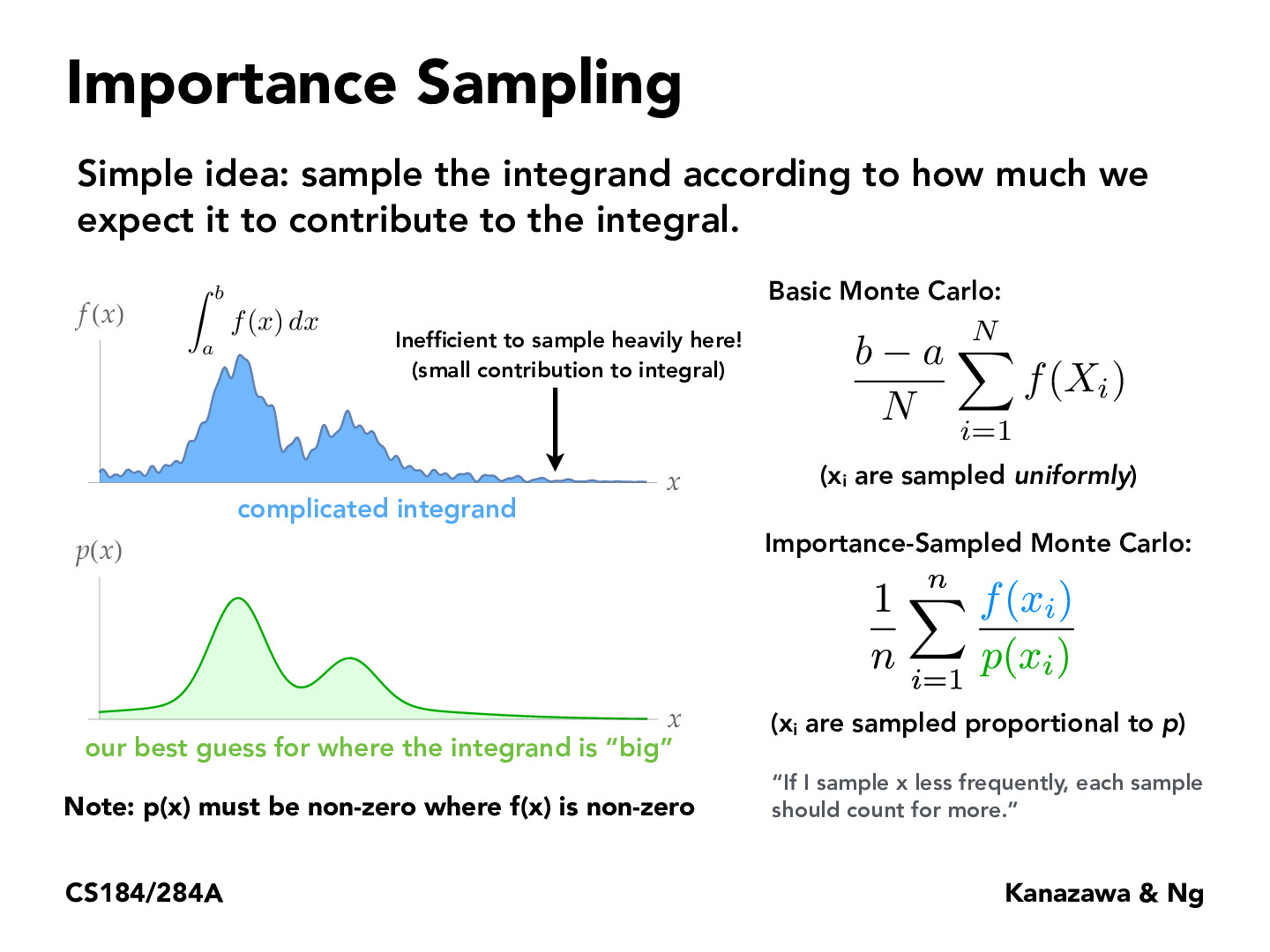Lecture 12: Monte Carlo Integration (38)bveeramani

I wonder how you would algorithmically generate p(x) from f(x). I figure it's probably challenging if you don't have the entire distribution f(x) a priori (you might only have the ability to evaluate f(x) at arbitrary points).

I read the Wikipedia article on Monte-Carlo integration, and it mentions a Metropolis-Hastings algorithm. It seems like the algorithm allows us to randomly sample from p(x) even if we only have the ability to evaluate f(x). So, I guess that's the answer to my question.wcyjames

If I understand it correctly, in practice when we sample f(x), we often expect such p(x) exists and this p(x) will give us a lower variance?herojelly

Maybe we could generate an approximate distribution for p(x) by making a bunch of buckets for values of x, sampling a bunch of points from f(x) for x values in each bucket, and counting the f(x) value of points from each bucket? I'm suggesting buckets because technically the probability that we get a specific x is 0 if the distribution is continuous.han20192019

I'm not sure if I understand correctly but I think we can still get a whole pdf for p(x)? It's just that the function for p(x) is not completely same as f(x).PongsatornChan

With p(x) like this, wouldn't this be biased sampling?dominicmelville

@PongsatornChan I have the same question. From what I can tell, as the general Monte Carlo estimator is probably unbiased, regardless of what the PDF is (see slide 25), then it doesn't matter if we use a constant PDF or one that weights by importance. However, this would also imply that a PDF which has weight 1 at a single location and weight 0 everywhere else would also result in an unbiased estimator, which doesn't make sense to me.ZizhengTai

Instead of importance sampling, does it make sense to sample more in the high-frequency regions (regions with the largest magnitude of changes within a certain window of x)? I'm thinking to have a sliding window to have a first pass over f(x) to find out these regions, and then do a second pass of actual sampling.daniswords

You can prove that importance sampling monte carlo is still unbiased by conducting a similar proof to slide 27. thats because we are concerned about expected value. The difference here is that importance sampling may have difference variance, but variance is not relevant to the proof.anthonytongUCB

This seems somewhat like circular logic? If our expectation of importance is accurate, then our sampling would be more accurate. So the closer our expectation is to the reality, the better our sampling is, but at that point we already must have a good idea of the integral.

You must be enrolled in the course to comment